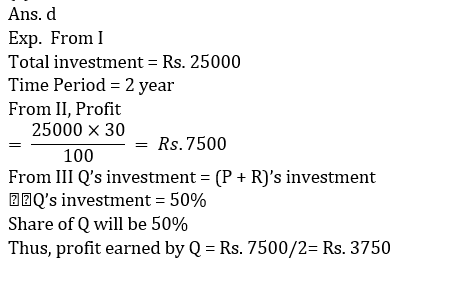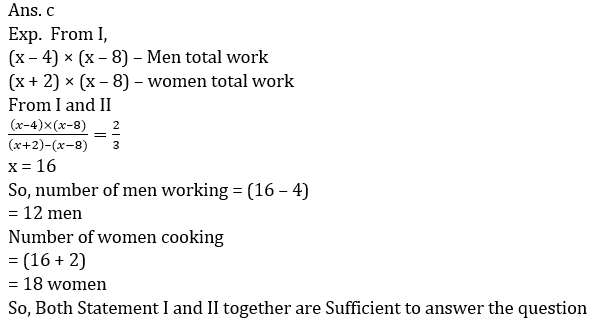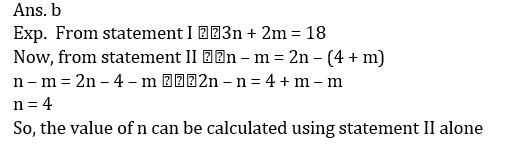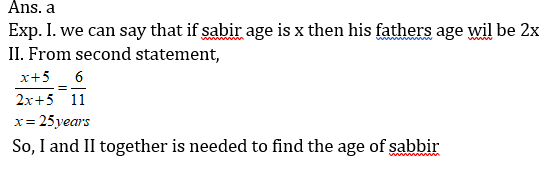# Data sufficiency Quiz for RRB PO & RRB CLERK : Quiz – 10

## Data sufficiency Quiz for RRB PO & RRB CLERK : Quiz – 10

Many competitive exams have Data Sufficiency Questions; therefore, you can’t take the risk of leaving out it. Answering Data Sufficiency Questions is tough for many applicants. If you practice more and more questions of Data Sufficiency Quiz, it will turn out to be simple for you. So, we have come up with Data Sufficiency Quiz to help you prepare for upcoming examinations such as banking, insurance, SSC, and railway. This Data Sufficiency Quiz is available for free to aspirants. Each question in this Data Sufficiency Quiz has a full explanation. This Data Sufficiency Quiz is based on the latest or updated exam pattern.

Directions (1 – 10): Each question below is followed by two or three statements I, II, and III. You have to determine whether the data given in the statements are sufficient for answering the question. You should use the data and your knowledge of Mathematics to choose between the possible answers. Read the statements and Answer.

1. What will be the share of Q in the profit earned by P, Q and R together?
I. P, Q and R invested total amount of Rs. 25000 for a period of two years.
II. The profit earned at the end of two years is 30%.
III. The amount invested by Q is equal to the amount invested by P and R together.
Only I
(b) Only II
(c) Only III
(d) All I, II and III are required to answer the question.
(e) None of these2. What will be profit share of Ankit out of total profit?
(I) Ankit Invest Rs. 1600 for 8 months and Satish joined him after third month with capital of Rs. 1200.
(II) Satish got Rs. 630 as profit share.
(a) Statement (I) alone is sufficient to answer the question but statement (II) alone is not sufficient to answer the questions.
(b) Statement (II) alone is sufficient to answer the question but statement (I) alone is not sufficient to answer the question.
(c) Both the statements taken together are necessary to answer the questions, but neither of the statements alone is sufficient to answer the question.
(d) Either statement (I) or statement (II) is sufficient to answer the question.
(e) Statements (I) and (II) together are not sufficient to answer the question.3. How many men and women working on this task?
(I) (x – 4) man work for first (x – 8) days, after that (x+2) woman complete the remaining work in (x – 8) days.
(II) Ratio between work did by men to work did by women is 2 : 3.
(a) Statement (I) alone is sufficient to answer the question but statement (II) alone is not sufficient to answer the questions.
(b) Statement (II) alone is sufficient to answer the question but statement (I) alone is not sufficient to answer the question.
(c) Both the statements taken together are necessary to answer the questions, but neither of the statements alone is sufficient to answer the question.
(d) Either statement (I) or statement (II) is sufficient to answer the question.
(e) Statements (I) and (II) together are not sufficient to answer the question.4. Find the cost price of laptop if cost price of laptop on both shops is same?
(I) Marked price of laptop on two shops A and B is same. Shops ‘A’ allows 20% discount and shop B allows 25% discount on laptop. A man purchased laptop from shop B, if man spend Rs. 640 more, he could purchase the same laptop from shop A.
(II) Marked price of laptop is 1100/21 % more than its cost price. If discount of 30% allows on M.P. of laptop, shopkeeper made a profit of Rs. 560.
(a) Statement (I) alone is sufficient to answer the question but statement (II) alone is not sufficient to answer the questions.
(b) Statement (II) alone is sufficient to answer the question but statement (I) alone is not sufficient to answer the question.
(c) Both the statements taken together are necessary to answer the questions, but neither of the statements alone is sufficient to answer the question.
(d) Either statement (I) or statement (II) is sufficient to answer the question.
(e) Statements (I) and (II) together are not sufficient to answer the question.5. There are some red and some blue balls in a bag. Find how many blue balls in the bag.
(I) Total number of balls in bag is ten. If selecting two balls at random probability of being at least one ball red is 14/15.
(II) Total number of balls in bag is ten. Selecting two balls from out of total balls at random,
probability of being both ball blue is 1/15 .
(a) Statement (I) alone is sufficient to answer the question but statement (II) alone is not sufficient to answer the questions.
(b) Statement (II) alone is sufficient to answer the question but statement (I) alone is not sufficient to answer the question.
(c) Both the statements taken together are necessary to answer the questions, but neither of the statements alone is sufficient to answer the question.
(d) Either statement (I) or statement (II) is sufficient to answer the question.
(e) Statements (I) and (II) together are not sufficient to answer the question.So, either statement I or II sufficient to give the answer of the question

6. What is the value of n?
I. 3n + 2m = 18
II. n – m = 2n – (4+m)
Only I
Only II
Both I and II
(d) Either I or II
(e) Cannot be determined7. Find out the length of train A.
I. Train A crosses another train B moving in the opposite direction in 10 sec.
II. Ratio of the speeds of trains A and B is 1 : 2.
III. Length of train B is 25% more than that of train A.
(a) All the three together are not sufficient
(b) Only I and II together
(c) All the three together
(d) Only I and II together
(e) Only II and III together8. What is the present age of Sabir?
I. The present age of Sabir is half of his father’s age.
II. After five years, the ratio of ages of Sabir and his father is 6 : 11.
III. Sabir is younger to his brother by five years.
(a) I and II
(b) I and III
(c) II and III
(d) All of these
(e) Cannot be determined9. Is 1/x greater than 1/y?
I. x is greater than 1.
II. x is less than y.
Only I
(b) Only II
(c) Both I and II
(d) Either I or II
(e) Cannot be determined#### Attempt Quantitative Aptitude Topic Wise Online Test Series

Recommended PDF’s for:

#### Most important PDF’s for Bank, SSC, Railway and Other Government Exam : Download PDF Now

AATMA-NIRBHAR Series- Static GK/Awareness Practice Ebook PDF Get PDF here
The Banking Awareness 500 MCQs E-book| Bilingual (Hindi + English) Get PDF here
AATMA-NIRBHAR Series- Banking Awareness Practice Ebook PDF Get PDF here
Computer Awareness Capsule 2.O Get PDF here
AATMA-NIRBHAR Series Quantitative Aptitude Topic-Wise PDF Get PDF here
AATMA-NIRBHAR Series Reasoning Topic-Wise PDF Get PDF Here
Memory Based Puzzle E-book | 2016-19 Exams Covered Get PDF here
Caselet Data Interpretation 200 Questions Get PDF here
Puzzle & Seating Arrangement E-Book for BANK PO MAINS (Vol-1) Get PDF here
ARITHMETIC DATA INTERPRETATION 2.O E-book Get PDF here
3